(Projected) Point on Line (2D) Algorithm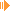Download Article and Source (70 kb)

Introduction

This little article presents different algorithms about the relation of a given point to a given line in the two-dimension case. In detail, following topics are addressed:

In every case, we have following input data:

• two points v1 and v2 which define the line to against. In particular, this implies that both points lie on this line of course.
• a third point p which acts as the test point.
• the vector from v1 to v2 is named e1. The length of e1 is denoted as |e1|.
• the vector from v1 to p is named e2. The length of e2 is denoted as |e2|.

1. Is test point on a line using y-intercept form ?

In this chapter it is tested if the given point p lies on the line using the y-intercept form which is well-known from school :-) This form defines a line using its slope (against the x-coordinate) and the intersection with the y-coordinate (y-intercept):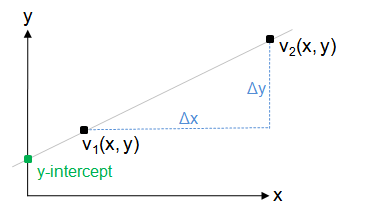The general equation of a line is y = m*x + b where m is the slope (defined as Δy/Δx) and b the y-intercept. As the line is given by two points on it, one can easily derive m: m = dy / dx = v2.y - v1.y / v2.x - v1.x

Having calulcated the slope, it is now used to derive the y-intercept by just inserting one of the two points (here v1 is used, but could be also interchanged with v2):

b = v1.y - m * v1.x

The line to test against is now completely defined by the y-intercept form. To check if the test point p lies on it, this equation can be easily used.
By putting the coordinate of the testpoint into the equation and using the actual line slope m, the y-intercept of test point p called b(p) should be equal to that of the line in case the test point lies on the line:

b(p) = p.y - m * p.x

If b(p) = b, the pt lies on the line.

Drawbacks:

However, this approach has some disadvantages:
• The most significant disadvantage: The x-intercept form cannot represent all lines. It is not possible to define a vertical line because it's slope would be infinite. This case would have to be handled in a special which makes this approach quite unattrative (note however that this case can be easily identified: the x-coordinate of both points are equal for vertical lines.)
• Another point to think about is the precision of floating point numbers. bt would never be perfectly equal to b, so an epsilon value must be used when testing for equality. However, finding such a epsilon is not easy and depends e.g. on the slope because the calculation error increaes with a larger slope, especially if the line converges to the vertical case.

2. Is point on a line using the perp dot product ?

The perp dot product provides a more better way for solving. Recall the perp dot product (PDP) of two two-dimensional vector e1 = (e1.x, e1.y) and e2 = (e2.x, e2.y) is defined as

perpDotProduct(e1, e2) = e1x * e2y - e1y * e2x

and actually calculates the area of the parallelogram spanned by the two vectors e1 and e2 as shown in the diagram below: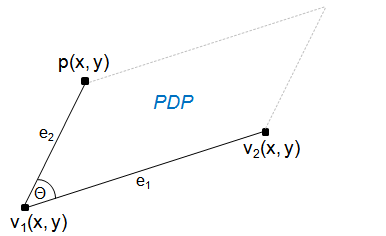Particularly, this implies that the pdp is 0 if both vectors e1 and e2 are parallel. If the two vectors are defined by the three points v1, v2 and p as shown in the figure above, it results obviously in the fact that the pdp is zero only if the p lies on the line e1 = vector from v1 to v2.

 /** * Calculates the area of the parallelogram of the three points. * This is actually the same as the area of the triangle defined by the three points, multiplied by 2. * @return 2 * triangleArea(a,b,c) */ float perpDotProduct(Point a, Point b, Point c) {   return (a.x - c.x) * (b.y - c.y) - (a.y - c.y) * (b.x - c.x); }

Again, there is the floating-point accuraccy problem, so instead of checking against zero, some epsilon value has to be used. Because the size of the pdp value depends on the length of the vectors, no static value can be used. One approach is to compare the pdp/area value to the fraction of another area which also depends on the length of the line e1=(v1, v2), e.g. the area of the square with side e1 which is computed below in function getEpsilon():

 public double getEpsilon() {   int dx1 = v2.x - v1.x;   int dy1 = v2.y - v1.y;   double epsilon = 0.003 * (dx1 * dx1 + dy1 * dy1);   return epsilon; }

In conclusion, the check if the point p lies on line v1v2 is then:

 boolean isPointOnLineviaPDP() {   return ( Math.abs(perpDotProduct(v1, v2, p) < getEpsilon()) ); }

3. Is point on a line segment using the perp dot product ?

The previous chapter explained how to test if the test point p lies on the given line in general, but not if it's on the closed line segment between both points in particular.
However, this turns out to be quite simple: The test point must lie inside the bounding box spanned by the two line points. Look at the figure below: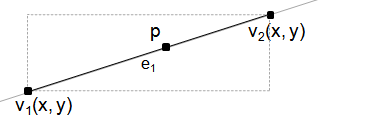The x-coordinate of the test point must lie in between the x-coordinates of both line points:

(v1.x <= p.x && p.x <= v2.x) || (v2.x <= p.x && p.x <= v1.x)

Note that '<=' is used instead of '<' to include also the endpoints of the line segment. The very same is also done for the y-coordinates:

(v1.y <= p.y && p.y <= v2.y) || (v2.y <= p.y && p.y <= v1.y)

In conclusion, the test point lies on the line segment if it lies on the general line (using algorithm of chapter 2) and it the test point is inside the bounding box. Below is the complete function to the line segment test:

 /** * Check if the point is on the line segment. */ public boolean isPointOnLineSegmentViaCrossProduct() {   if (!( (v1.x <= p.x && p.x <= v2.x) || (v2.x <= p.x && p.x <= v1.x) ))   {     // test point not in x-range     return false;   }   if (!( (v1.y <= p.y && p.y <= v2.y) || (v2.y <= p.y && p.y <= v1.y) ))   {     // test point not in y-range     return false;   }   return isPointOnLineviaPDP(); }

4. Is the projection of a point on a line segment using the perp dot product ?

In this chapter an algorithm is presented to test if the projected point p' of the point p onto the line e1 lies on inside the closed line segment.
The projected point p' is the nearest point to p that lies on the given line. Have a look at the figures below: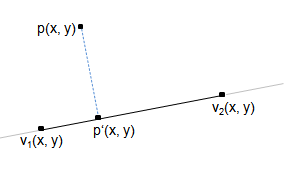Projected point p' is on line segment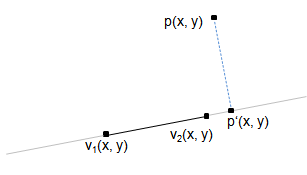Projected point p' is not on line segment

In the left figure, the projected point p' lies inside the line segement, while this is not the case in the right figure.
To handle this problem, we use the dot product this (not the perp dot product!).

Small excursion:

The dot product (DP) and perp dot product (PDP) are related, but are not identical.
Both calculate a scalar value from two vectors. However, the perp dot product is obtained by calculating the dot product of one vector with the perpendicular vector (the one that is 90 degrees rotated) of the other one. Direct comparison of equations:

PDP(v1, v2) = vx1 * vy2 - vy1 * vx2 = |e1| * |e2| * sin(α)

DP(v1, v2) = vx1 * vx2 + vy1 * vx2 = |e1| * |e2| * cos(α)

where |v1|, |v2| are the length of the vector and α is the angle between both vectors.
Because the perpendicular vector v' of a vector v (x1, y1) is v'(-y1, x1), the derived definition of PDP from DP is obvious.

Let's get back to the actual problem:
Consider the vectors e1 = (v2, v1) and e2 = (pv1).

• If p' matches with v1 (this means both have the same location), then
DP(e1, e2) = 0. e1 is then perpendicular to e2.
• On the other side, if p' matches with v2 (thus p' is the same as v2), then
DP(e1, e2) = DP(e1, e1) = e1.x^2 + e1.y^2.
• If p' lies somewhere between v1 and v2, then for the dot-product of e1 and e2 applies:
0 <= DP(e1,e2) <= e1.x^2 + e1.y^2.

Here follows the code:

 /** * Check if the projected testpoint onto the line is on the line segment. * @return true if projected point is on line segment, false otherwise */ public boolean isProjectedPointOnLineSegment() {   // get dotproduct |e1| * |e2|   Point e1 = new Point(v2.x - v1.x, v2.y - v1.y);   int recArea = dotProduct(e1, e1);   // dot product of |e1| * |e2|   Point e2 = new Point(p.x - v1.x, p.y - v1.y);   double val = dotProduct(e1, e2);   return (val > 0 && val < recArea); }

5. What are the coordinates of the projected point on a line segment using the perp dot product ?

In the previous chapter it was only checked if the projected point p' lies on the line segment v1v2, however the actual coordinates were not calculated. This is now caught up in this final section!

Let's have a look at the following figure: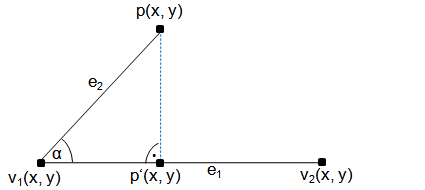At first let's calculate angle α using the dot product. We know that DP(e1, e2) = |e1| * |e2| * cos(α). As we know all three points and so also the vectors e1 and e2, it's easy to get cos(α):

cos(α) = DP(e1, e2) / (|e1| * |e2|)

Because the line pp' (blue line in the figure) is perpendicular to e1, cos(α) is also defined as cos(α) = |v1p'| / |e2|, so we can get the length of the line segment from v1 to p':

|v1p'| = cos(α) * |e2|

As the points v1 and v2 are known as well as the distance from v1 to p', we starting from v1 we can just 'go along' to p'. In particular, the position of p' is can be calculated as:

p'.x = v1.x + ((|v1p'| / |e1|) * e1.x)
p'.y = v1.y + ((|v1p'| / |e1|) * e1.y)

Here the code of the whole function:

 /** * Get projected point P' of P on line e1. * @return projected point p. */ public Point getProjectedPointOnLine() {   // get dot product of e1, e2   Point e1 = new Point(v2.x - v1.x, v2.y - v1.y);   Point e2 = new Point(p.x - v1.x, p.y - v1.y);   double valDp = dotProduct(e1, e2);   // get length of vectors   double lenLineE1 = Math.sqrt(e1.x * e1.x + e1.y * e1.y);   double lenLineE2 = Math.sqrt(e2.x * e2.x + e2.y * e2.y);   double cos = valDp / (lenLineE1 * lenLineE2);   // length of v1P'   double projLenOfLine = cos * lenLineE2;   Point p = new Point((int)(v1.x + (projLenOfLine * e1.x) / lenLineE1),                       (int)(v1.y + (projLenOfLine * e1.y) / lenLineE1));   return p; }

Be inspecting this calculation, a simplification is possible. Rechecking the calculation of variables cos, projLenOfLine and final calculation of p'.x and putting it altogether, we get following (example for x-coordinate, analogous for the y-coordinate):

p'.x = (valDp / (lenLineE1 * lenLineE2)) * lenLineE2 * e1.x / lenLineE1

Calculating the length of the lines e1 and e2 is quite expensive due to the square root.
However, the equation above deptics that lenLineE2 cancels out itself. Furthermore, the calculation contains lenLineE1 * lenLineE1 which also removes the sqaure root and is simply: e1.x * e1.x + e1.y * e1.y. These facts are used in the optimized version of getProjectedPointOnLine() below:

 /** * Get projected point P' of P on line e1. Faster version. * @return projected point p. */ public Point getProjectedPointOnLineFast() {   // get dot product of e1, e2   Point e1 = new Point(v2.x - v1.x, v2.y - v1.y);   Point e2 = new Point(p.x - v1.x, p.y - v1.y);   double valDp = dotProduct(e1, e2);   // get squared length of e1   double len2 = e1.x * e1.x + e1.y * e1.y;   Point p = new Point((int)(v1.x + (val * e1.x) / len2),                       (int)(v1.y + (val * e1.y) / len2));   return p; }

That's it. Hope you enjoyed this article and will find the applet + source useful!

Sunshine, December 2013

This site is part of Sunshine's Homepage